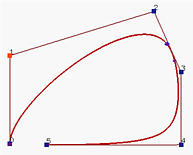# NURBS Curves: Knot InsertionSince NURBS curves are the projections of 4D B-spline curves to 3D, knot insertion for NURBS curves is easy. In fact, knot insertion for NURBS curves is done in three steps: (1) converting the given NURBS curve in 3D to a B-spline curve in 4D, (2) performing knot insertion to this four dimensional B-spline curve, and (3) projecting the new set of control points back to 3D to form the the new set of control points for the given NURBS curve.

Suppose we have n + 1 control points P0, P1, ..., Pn with associated weights w0, w1, ..., wn, a knot vector U and a degree p. Let Pi = (xi, yi, zi). Then, control points Pwi = ( wixi, wiyi, wizi, wi ), 0 <= i <= n, and knot vector U define a four dimensional B-spline of degree p. The insertion of a new knot t to this four dimensional B-spline yields a new set of control points Qwi = ( Xi, Yi, Zi, Wi ), 0 <= i <= n. Projecting them back to the three-dimensional space by dividing the first three components with the fourth yields the new set of control points of the given NURBS curve.

Let us take a look at an example. Suppose we have 9 knots

 u0 to u3 u4 u5 to u8 0 0.5 1

and a NURBS curve of degree 3 defined by the following 5 control points in the xy-plane:

 x y w P0 -70 -76 1 P1 -70 75 0.5 P2 74 75 4 P3 74 -77 5 P4 -40 -76 1

The following shows the curve and its basis functions.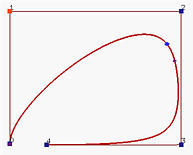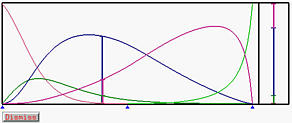Let us insert a new knot t = 0.4. Since t is in knot span [u3, u4) and the degree of the NURBS curve is 3, the affected control points are P3, P2, P1 and P0. Since this is a NURBS curve, we shall use homogeneous coordinates by multiplying all control points with their corresponding weights. Call these new control points Pwi:

 x y w Pw0 -70 -76 1 Pw1 -35 37.5 0.5 Pw2 296 300 4 Pw3 370 -385 5

Note that since P4 is not affected, it is not computed in the above table. Then, we shall compute a3, a2 and a1 as follows: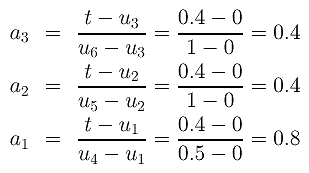The new control points Qw3, Qw2 and Qw1 are: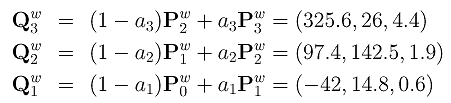Projecting these control points back to 2D by dividing the first two components with the third (the weight), we have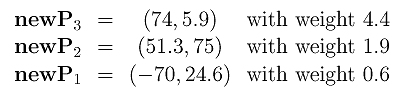The following is the resulting NURBS curve and its basis functions: